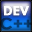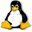Home Latest News Archive Tutorials C++ 2 3 4 Win32 Java OpenGL GLUT OpenGL ES 2 3 4 DirectX VB.NET HTML Photoshop 3dsmax Forums Projects Modelling Links About Us Search Recent Tutorials C / C++ Win32 OpenGL OpenGL ES GLUT## Dynamic Arrays

Tutorials > C++ > Dynamic Arrays

### Introduction

You may have noticed that you cannot create an array unless the exact size is known.
If you tried to create an array eg. int nums, all would be fine. If however, you tried to create an array eg. int nums[i], you would get errors.

The array above is known as a dynamic array. If you would like to create a dynamic array, you need to allocate memory as was shown in the previous tutorial.

Contents of main.cpp :

```#include <iostream>
#include <stdlib.h>
#include <fstream>

using namespace std;

int main()
{
```

Below we declare 2 pointers to integers. We have dealt with pointers to an integer before. A pointer can also point to the first integer in an array of values.

```	int *cnums = NULL;
int *cppnums = NULL;
```

The code below opens a text file. The first line of the file indicates how many numbers are in the array and each successive line holds a number to place in the array.

```	int numNums;

ifstream fin("in.txt");

fin >> numNums >> ws;
```

In C, you use the malloc function, but instead of specifying the amount of memory to allocate as sizeof(int), you specify the amount of bytes as the size of the data type multiplied by the amount of items you want in the array.

```	// C Dynamic Array
//-----------------
cnums = (int *)malloc(sizeof(int) * numNums);
```

In C++, you still use the new keyword followed by the data type that you are wanting to create the array for, but this time you place square brackets containing the size of the array. The size of the array is given as how many items you would like, not the number of bytes.

```	// C++ Dynamic Array
//-------------------
cppnums = new int[numNums];
```

The arrays can now be used as per normal.

```	int temp = 0;
for (int i = 0; i < numNums; i++)
{
fin >> temp >> ws;

cnums[i] = temp;
cppnums[i] = temp;
}

fin.close();

for (int i = 0; i < numNums; i++)
cout << "c : " << cnums[i]
<< " cpp : " << cppnums[i] << endl;
```

Arithmetic can also be done on pointers. If you add 2 to the cppnums array and dereference the pointer position to access the data, you will receive 87, being the number in position 2.

```	cout << *(cppnums + 2) << endl;
```

In C, there is no change in how you deallocate the memory previously allocated. You simply use the free function and pass the pointer as a parameter.

```	// C Release of Array
//--------------------
free(cnums);
```

In C++, the deallocation mechanism is slightly different. When you are reclaiming memory from an array, you need to place square brackets straight after the delete keyword. This indicates that an entire array must be deallocated. If you left out these square brackets, only the memory for the first item would be released.

```	// C++ Release of Array
//----------------------
delete[] cppnums;

system("pause");

return 0;
}```

Well done. You should now be able to create dynamic arrays. This is very useful as you will normally find that you do not know the size of an array at compile time.

Please let me know of any comments you may have : Contact Me

 Source Files :< Tutorial 40 - Memory Allocation Tutorial 42 - Dynamic Matrices >